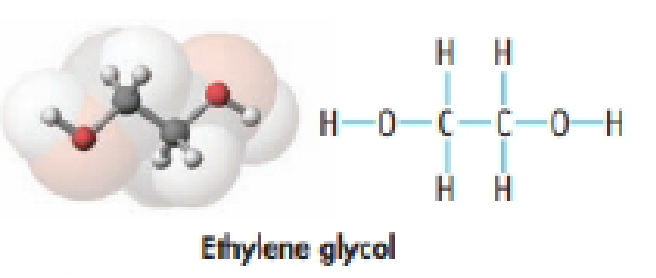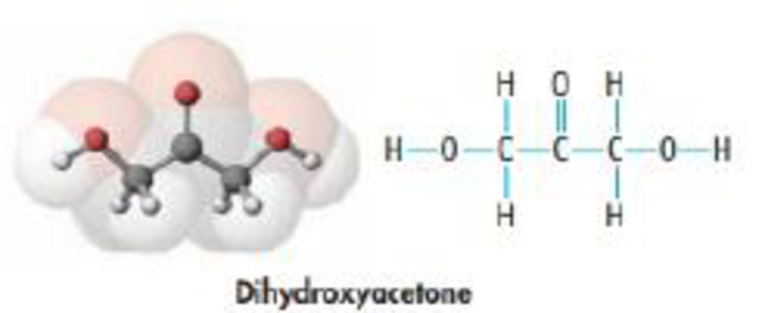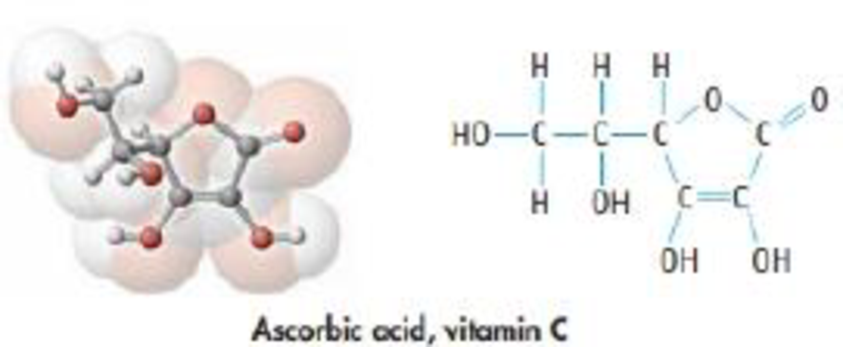Chapter 2, Problem 124GQ

Chapter
Section
Textbook Problem

Write the molecular formula and calculate the molar mass for each of the molecules shown here. Which has the largest mass percent of carbon? Of oxygen?(a) ethylene glycol (used in antifreeze)(b) dihydroxyacetone (used in artificial tanning lotions)(c) ascorbic acid, commonly known as vitamin CInterpretation Introduction

Interpretation: Molar mass and molecular formula of the given compound should be determined and the compound which has the largest mass percent of carbon and oxygen also has to be determined.

Concept Introduction:

• Moleofsubstance=GramofsubstanceMolarmass
• A chemical formula conveys us the number of atoms of each element in a compound. It contains the symbols of the atoms of the elements present in the compound as well as how many are for each element in the form of subscripts
• The molar mass of an element or compound is the mass in grams of 1 mole of that substance, and it is expressed in the unit of grams per mol (g/mol)
• Mass percentage is one way to represent the concentration of an element in a compound or a component in a mixture. Mass percent of elements of a compound is the ratio of mass of element to the mass of whole compound and multiplied with hundred.
Explanation

Given,

Compound a is,

There are two carbon atoms, two oxygen atoms and six hydrogen atoms

Then molecular formula of this compound is C2H6O2

Molar mass of ethylene glycol can be calculated as follows,

(12.01massofC)+(1massofH)+(16massofO)=62.02g/mol

Given

Compound b is,

There are three carbon atoms, three oxygen atoms and six hydrogen atoms

Then molecular formula of this compound is C3H6O3

Molar mass of dihydroxyacetone can be calculated as follows,

(12.01massofC)+(1massofH)+(16massofO)=90.03g/mol

Similarly,

The given structure of compound c is,

There are six carbon atoms, six oxygen atoms and eight hydrogen atoms.

Then molecular formula of this compound is C6H8O6

Molar mass of ascorbic acid can be calculated as follows,

(12.01massofC)+(1massofH)+(16massofO)=176.06g/mol

Mass percent of elements of a compound is the ratio of mass of element to the mass of whole compound and multiplied with hundred.

The molecular formula of the given compound a is C2H6O2 and its molar mass is 62.02g/mol

And this compound namely Ethylene glycol contains two carbon atoms, molar mass of carbon atom is 12.01g/mol

Molar mass of carbon in compound is 2×12.01=24.02g/mol.

MasspercentofCarboninC2H6O2=MolarmassofcarbonMolarmassofC2H6O2×100 =24.0262.02×100 =38.72%

Similarly, Molar mass of oxygen in compound 2×16=32g/mol

MasspercentofoxygeninC2H6O2=MolarmassofoxygenMolarmassofC2H6O2×100 =3262.02×100 =51.6%

The molecular formula of the given compound b is C3H6O3 and its molar mass is 90

Still sussing out bartleby?

Check out a sample textbook solution.

See a sample solution

The Solution to Your Study Problems

Bartleby provides explanations to thousands of textbook problems written by our experts, many with advanced degrees!

Get Started